# Samacheer Kalvi Books: Tamilnadu State Board Text Books Solutions

## Samacheer Kalvi 8th Maths Chapter 7 Information Processing Notes PDF Download: Tamil Nadu STD 8th Maths Chapter 7 Information Processing NotesSamacheer Kalvi 8th Maths Chapter 7 Information Processing Notes PDF Download: Tamil Nadu STD 8th Maths Chapter 7 Information Processing Notes

## Samacheer Kalvi 8th Maths Chapter 7 Information Processing Notes PDF Download

We bring to you specially curated Samacheer Kalvi 8th Maths Chapter 7 Information Processing Notes PDF which have been prepared by our subject experts after carefully following the trend of the exam in the last few years. The notes will not only serve for revision purposes, but also will have several cuts and easy methods to go about a difficult problem.

 Board Tamilnadu Board Study Material Notes Class Samacheer Kalvi 8th Maths Subject 8th Maths Chapter Chapter 7 Information Processing Format PDF Provider Samacheer Kalvi Books

## How to Download Samacheer Kalvi 8th Maths Chapter 7 Information Processing Notes PDFs?

2. Click on the Samacheer Kalvi 8th Maths Notes PDF.
3. Look for your preferred subject.
4. Now download the Samacheer Kalvi 8th Maths Chapter 7 Information Processing notes PDF.

## Tamilnadu Samacheer Kalvi 8th Maths Solutions Chapter 7 Information processing InText Questions

Recap (Text Book Page No. 232 & 233)

Question 1.
Find the number of all possible triangles that can be formed from the triangle given below.Answer:
Single Triangles ⇒ 5
Combination of 2 ⇒ 4
Combination of 3 ⇒ 2
Big triangle ⇒ 1
= 12

Question 2.
Use the numbers given in the figure to form a 3 × 3 magic square.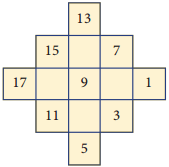Question 3.
Convert the tree diagram into a numeric expression.(10 × 5) + (9 × 4)
= 50 + 36
= 86

Question 4.
(i) Find the total time taken by the bus to reach from A to E via B , C and D.
(ii) Find which is the shortest route from A to E.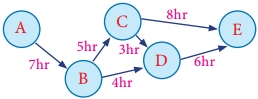(i) Route ⇒ A → B → C → D → E
Time taken ⇒ (7 + 5 + 3 + 6) hrs = 21 hrs

(ii)

 Available roots Time taken (a) A → B → C → D → E 7 + 5 + 3 + 6 = 21 hrs (b) A → B → D → E 7 + 4 + 6  = 17 hrs (c) A → B → C → E 7 + 5 + 8 = 20 hrs

Shortest route ⇒ A → B → D → E

Question 5.
Connect the Fibonacci squares through diagonals by curve from corner to corner across each square to draw a Golden Spiral.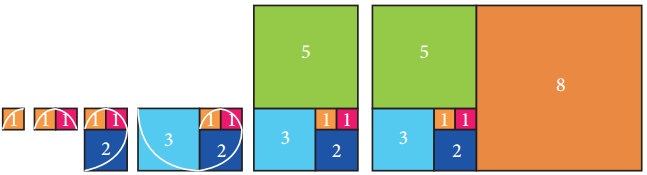Fibonacci Squares

Question 6.
When you plan to buy a shirt, one shop offers a discount of ₹ 200 on MRP ₹ 1000 and another shop offers 15% discount on the same MRP. Where would you buy?
Price in 1st shop = ₹ 1000 – ₹ 200 = ₹800In shop 1.

Question 7.
Amazing park is offers a package deal of 5 entrance passes for ₹ 130. If one entrance pass normally costs ₹ 30, how much will you save by taking advantage of this special deal?
Cost of one entrance pass = ₹ 30
∴ Cost of 5 entrance passes = ₹ 5 × 30
= ₹ 150
But special deal price = ₹ 130
Amount of saving = 150 – 130 = ₹ 20

Activity (Text Book Page No. 237)

Question 1.
Determine the number of two digit numbers that can be formed using the digits 1, 3 and 5 with repetition of digits allowed.
The activity consists of two parts
(i) Choose a one’s digit.
(ii) Choose a ten’s digit.
Complete the table given beside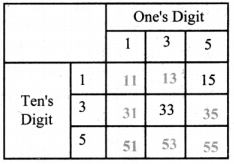Question 2.
Find the three digit numbers that can be formed using the digits 1, 3 and 5 without repetition of digits.
Complete the tree diagram given below to the numbers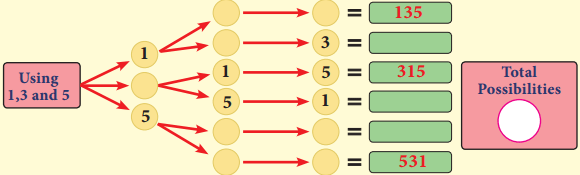Activity (Text Book Page No. 242)

Question 1.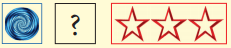(i)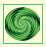(ii)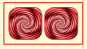(iii)Question 2.(i)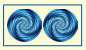(ii)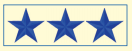(iii)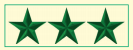Question 3.(i)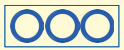(ii)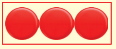(iii)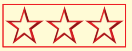Question 4.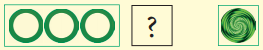(i)(ii)(iii)Question 5.(i)(ii)(iii)Try These (Text Book Page No. 243)

Question 1.
Find any five SETs among these set of cards (repetition of cards allowed).
a.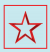b.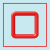c.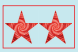d.e.f.g.h.i.j.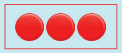(i) (a), (c), (h)
(ii) (e), (g), (j)
(iii) (a), (b), (d)
(iv) (f), (i), (j)
(v) (f), (i), (h)

Question 2.
This is an example for a magic square in SETs. Can you make another two?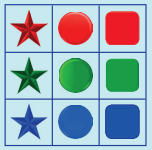Repeat one square with every thing 2 another square with everything 3.

Activity (Text Book Page No. 250)

Using the given Table I, find the pattern, answer the following questions and colour the values in the given Table Il. One is done for you.
Table – 1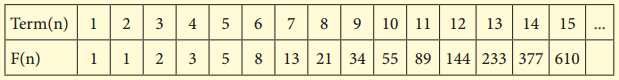Question 1.
Where are the even Fibonacci Numbers?
Colour both the term n and where F(n) is even in yellow.
Do you find any pattern?
Every Third Fibonacci number is a multiple of 2(even).
i.e., a multiple of F(3) or 2 = F(3)

Question 2.
Where there are Fibonacci numbers which are multiple of 3?
Colour both the term n and where F(n) is multiple of 3 in red.
Write down the pattern you find
Every 4th Fibonacci number is a multiple of 3.
i.e., a multiple of F(4) or 3 = F(4)

Question 3.
What about the multiple of 5?
Colour both the term n and where F(n) is multiple of 5 in blue. ‘
Write down the pattern you find.
Every …………………………… i.e ………………………………
Every 5th Fibonacci number i.e a multiple of F(5) or 5 = F(5)

Question 4.
What about the multiple of 8?
Colour both the term n where F(n) is multiple of 8 in green.
Write down the pattern you find.
Every …………………………… i.e ………………………………
Every 6th Fibonacci number i.e a multiple of F(6) or 8 = F(6)

Table 2.From the above activity, we conclude that
Every Fibonacci number is a factor of (a term number of) Fibonacci numbers in multiples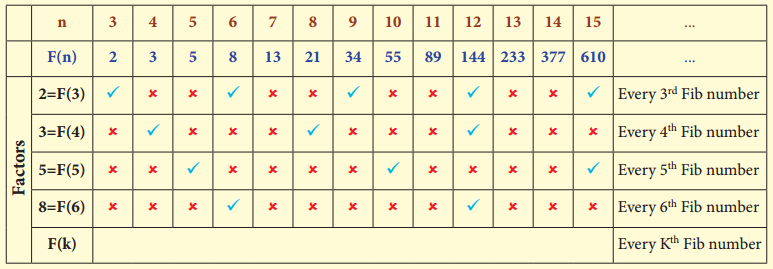From the above table, we get a general rule as Every kth Fibonacci number is a multiple of F(k).
Table 2.R – Red
Y – Yellow
B – Blue
G – Green

Activity (Text Book Page No. 258)

Code 1: Pigpen

I. Fill in the blank boxes and decode
The Pigpen code looks like meaningless writing, but it is quite easy to catch on to. Each letter is represented by the part of the “Pigpen” that surrounds it.
The first code uses the following key. To complete the code, you need to work out how to use the key to decode the message.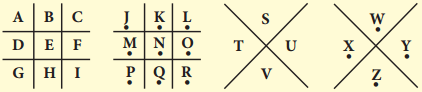To decodeCode 2: Polybius Square Cipher

II. Fill in the blanks
A Polybius Square is a table that allows someone to convert letters into numbers. Use the Polybius square rows and column values to find the codeUsing this a set of numbers can be used to denote a letter and vise versa
(4, 2) represent T, (3, 4) represent H, (5, 5) represent E
Likewise, when we solve for the remaining. we get
THE NAME OF TREASURE DOES NOT HAVE BAND D .
Since clue 2 gives that the treasure item does not have the letters b & d, therefore “exam pad” & “geometry box” cannot be the treasure item.
Hence, the treasure item is “gift voucher”

Code 3: Atbash Cipher
III. Find the code using the key as shown in given figure:
Atbash cipher is a substitution cipher with just one specific key where all the letters are reversed that is A to Z and Z to A.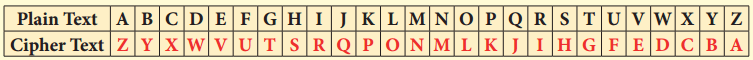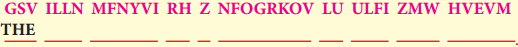The given Cipher is:
GSV ILLN MFNYVI RH Z NFOGRKOV LU ULFI ZMW HVEVM
Decoded Message:
THE ROOM NUMBER IS A MULTIPLE OF FOUR AND SEVEN
Since room number is a multiple of 4 & 7,
The room number is 4 × 7 = 28.

Code 4: Using a Key – Reflection Table
IV Use the reflection table which is given below and find the correct word by using a reflected alphabet.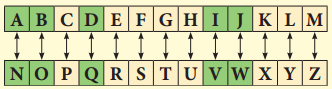J V A Q B J – _______
P B Z C H G R E G N O Y R – _______
P U N V E – ________
P H O O B N E Q – ________
J V A Q B J – WINDOW
P B Z C H G R E G N O Y R – COMPUTER TABLE
P U N V E – CHAIR
P H O O B N E Q – CUPBOARD

After finding the codes, the teacher then asks students to rearrange the clues one by one
CLUES
1. ————————————————-
2. ————————————————-
3. ————————————————-
4. ————————————————-
The 4 clues are:
2. The name of the treasure does not have b’ & ‘d’
3. The room is a multiple of four and seven – 28
4. Window, Computer table, Chair, Cupboard

RESULT
(i) The room in which the treasure took place = ________ .
(ii) The place of the treasure = ________ .
(iii) The identity of the treasure = ________ .
(Hint:- If you answered the question number 6 in Exercise 4.3, you can compare and verify your results) The gift voucher contains (20 full marks awarded).
The room in which the treasure took place = 28
The place of treasure = Chair
Identity of treasure = Gift voucher.

Try These (Text Book Page No. 260)

Question 1.
Use Pigpen Cipher code and write the code for your name _______ and your chapter names
(i) LIFE MATHEMATICS
(ii) ALGEBRA
(iii) GEOMETRY
(iv) INFORMATION PROCESSING
For Pigpen, we should first construct the Criss Cross pattern & then fill with alphabets.
Step 1: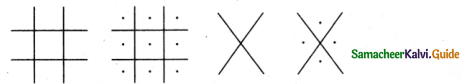Step 2: Fill the above with all the alphabets from A to Z.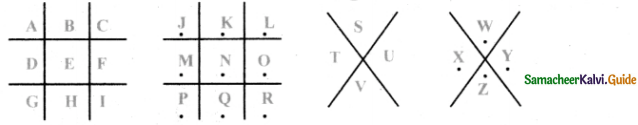Step 3: So, the secret code is got by the outline of each letter in the above patterns.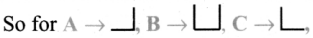similarly for all the others. Therefore pigpen code is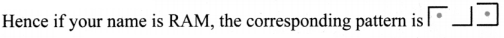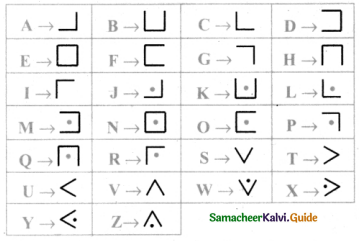Let us now write the given words with Pigpen code.Question 2.
Decode the following Shifting and Substitutmg secret codes given below. Which one is easier for you?
(i) Shifting method:- M N S G H M F HR H L O N R R H A K D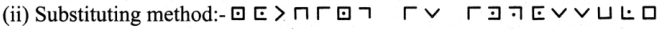Decode using shifting method. Let us shift & see by trial & error method.
Let us substitute ‘b’ for ‘a’, ‘e’ for ‘b’ & so on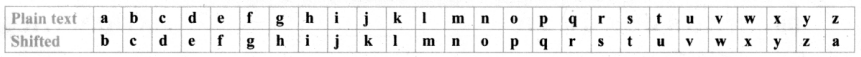Message : M N S G H M F H R H L O N R R H A K D
Decoded text : NOTHING IS IMPOSSIBLEWe find that Pigpen code has been used, by substituting for the symbols from the table that we constructed earlier, we go the message.
NOTHING IS IMPOSSIBLE

Question 3.
Write a short message to a friend and get them to decipher it.(Use any one shifting and substituting code method)
Student can choose and tr on their own.

Activity (Text Book Page No. 264)

Consider that you are going to a store with your total budget of ₹ 220 to buy things without changing the quantity of the items given in the list below with the following conditions.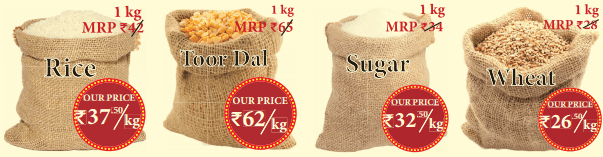Conditions:
(i) First you have to complete the price list given.
(ii) You have to buy three items as per the given price list but within your budget ₹ 220.
(iii) You wont carry exceeding 5kg because you have to walk home carrying them, so they cannot be bulky.

1. In how many ways can you buy your items? Complete the price lists given below. One is done for you.
2. Which one is the best purchase price list and why?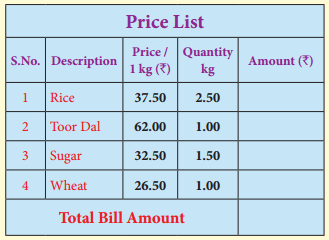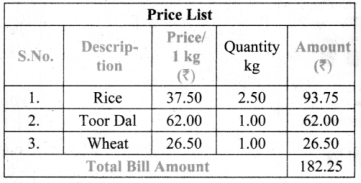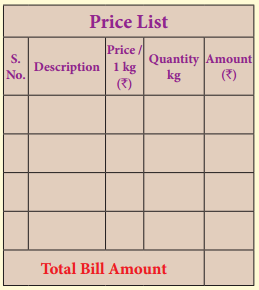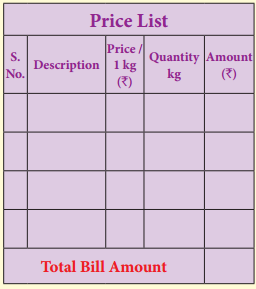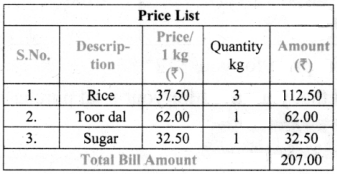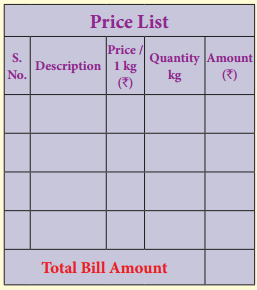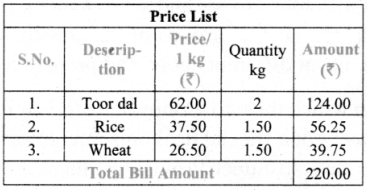Activity (Text Book Page No. 265)

Consider that you want to buy 12 litres of the same quality of edible oil at your budget price of ₹ 250 per litre. In a supermarket, there are a lot of offers on various oil brands. Some of the offers are given below. Complete the table and find which one is the best offer for you and how much you will save for your total purchase.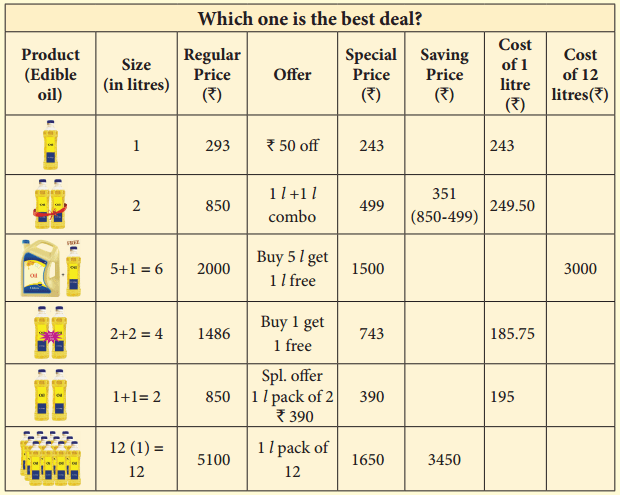Best offer price for you ___________
Amount that you saved for your total purchase ___________
Best offer price for you ₹ 137.50
Amount that you saved for your total purchase ₹ 1350

Try These (Text Book Page No. 266)

The teacher divides the class into four groups and setup a mock market in the class room and ask the students to involve in role play as Iwo groups of businessmen and two groups of consumers. Consumers have to buy products at different shops and prepare a price list.

The Iwo supermarkets iii which the two groups buy are Star Food Mart and Super Provisions. Th is week they each have got a special deal on some products. At Star Food Mart. you can buy items at discount prices. At Super Provisions, there are some “BUY ONE GET ONE” deals. Have a look at their deal: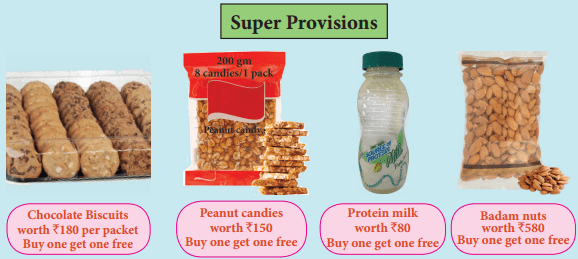I. Here is your shopping list:
4 bottles of Protein Milk (200 ml size) 2 packets of Peanut candies(200 gm), 1 packet of Chocolate biscuits, 1 packet of Badam nuts (500 gm)
price of Star Food Mart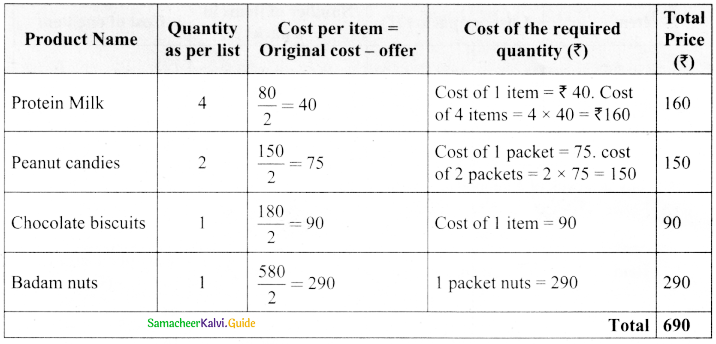In star Food Mart

(ii) If you buy the items from the two shops, how will you do it to spend the least amount of money?
Badam nuts from super provisions and other items from Star Food Mart.

II. You have H 000/- to spend to buy the following shopping list:
6 bottles of Protein Milk (200 ml size), 3 packets of Peanut candies(200 gm), 3 packets of Chocolate biscuits, 1 packet of Badam nuts (250 gm).
Comparing two stores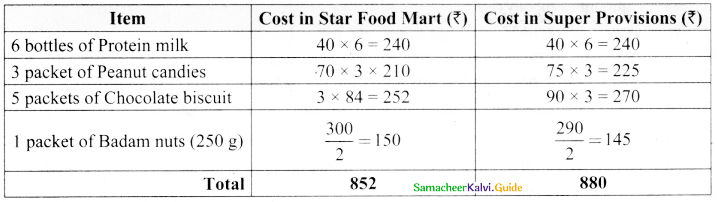(i) How can you do this so that you dont go over your budget amount ₹ 1000?
We can buy them from any one shop.

(ii) Which shop offers you the best value for money on each item?
Star Food Mart

(iii) Is the “BUY ONE GET ONE” deal at Super Provisions the same as “50% off” deal?
Yes

## How to Prepare using Samacheer Kalvi 8th Maths Chapter 7 Information Processing Notes PDF?

Students must prepare for the upcoming exams from Samacheer Kalvi 8th Maths Chapter 7 Information Processing Notes PDF by following certain essential steps which are provided below.

• Use Samacheer Kalvi 8th Maths Chapter 7 Information Processing notes by paying attention to facts and ideas.
• Pay attention to the important topics
• Refer TN Board books as well as the books recommended.
• Correctly follow the notes to reduce the number of questions being answered in the exam incorrectly
• Highlight and explain the concepts in details.

## Frequently Asked Questions on Samacheer Kalvi 8th Maths Chapter 7 Information Processing Notes

#### How to use Samacheer Kalvi 8th Maths Chapter 7 Information Processing Notes for preparation??

Read TN Board thoroughly, make separate notes for points you forget, formulae, reactions, diagrams. Highlight important points in the book itself and make use of the space provided in the margin to jot down other important points on the same topic from different sources.

#### How to make notes for Samacheer Kalvi 8th Maths Chapter 7 Information Processing exam?

Read from hand-made notes prepared after understanding concepts, refrain from replicating from the textbook. Use highlighters for important points. Revise from these notes regularly and formulate your own tricks, shortcuts and mnemonics, mappings etc.
Share: